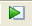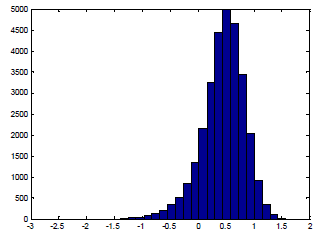# How to make probabilistic calculations with a Matlab script.

Recommended is to use the scripts developed by Kees den Heijer.  For background info is referred to:

den Heijer, C.  Reliability methods in OpenEarthTools, Communications on Hydraulic and Geotechnical Engineering 2012-01

You may download the script, including an example of a probabilistic calculation of armour stone for a breakwater or revetment with the Van der Meer formula. You can easily change the code for this example by any code you need for your specific problem. For details see above publications. For a quick start see the recipe below.

### Recipe:

1. Verify that Matlab is installed on your computer.
3.  Unzip this file in a directory on your computer.
4.  Double click on ….\oetsettings.m
Matlab will open and the script with the settings. When all is loaded, you run the program by clicking on the “run” icon in the command bar:5. “alpha” gives a list of α-values for all input variables (in the sequence of input), so in our (Van der Meer) case: ρs, ρw, tanα, steep, P, S, N, H, dn50, Cpl.
6. The value of Beta and the found failure probability is given
7. Matrix “designpoint” gives the values for the design point.
8. For MC you find
1. The found failure probability p_F. (realise that p_F is different in each computation)
2. In the matrix z you find all found z values (in our example 30000). You may make a histogram of this array. You can see how much samples were negative. This quantifies the failure from your MC experiment. The default command for the histogram is: >>hist(resultMC.Output.z(1:30000,1)); figure(gcf)9. By adding a number you can increase the amount of bins:
>>hist(resultMC.Output.z(1:30000,1),30); figure(gcf).
10. The matrix x gives you all the input parameters for all the 30000 MC computations made for this experiment. You also may plot them with the histogram option. This is sometimes useful to debug your program.